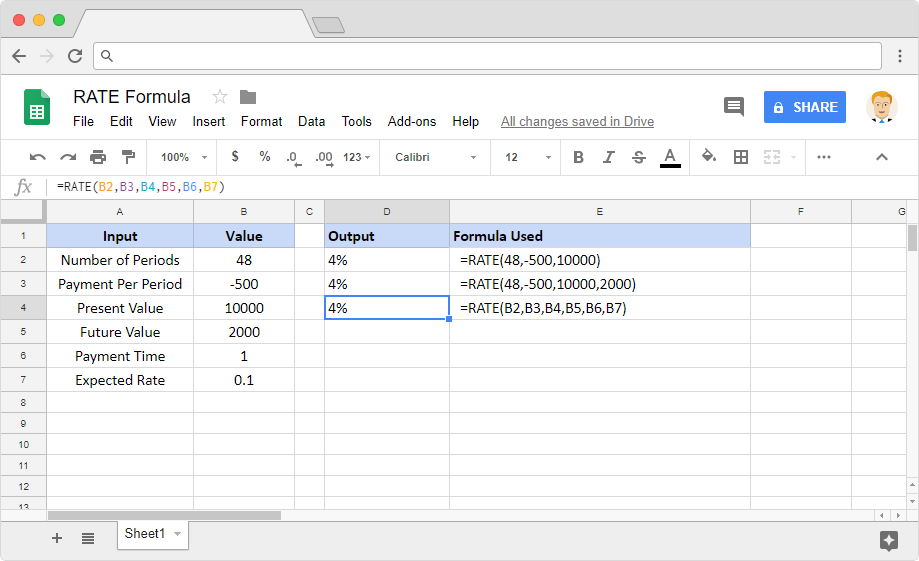# How to use the RATE formula in Google SheetsLet’s assume that we have a number of periodic constant-value payments to make against an investment. So naturally, we would be interested to know the underlying interest rate. How do we calculate it easily? Is there any ready-made tool available to help us with that? Yes, the RATE formula in Google Sheets is ported for this exact purpose.

### Syntax

RATE(number_of_periods, payment_per_period, present_value, [future_value, end_or_beginning, rate_guess])

• number_of_periods – is the number of periods that the buyer wishes to make payment for.
• payment_per_period – is the amount the buyer pays per period.
• present_value – is the current value of the annuity.
• future_value [ OPTIONAL ] – is the amount of the future value that remains after we make the final payment.
• end_or_beginning [ OPTIONAL, 0 by default ] – a value of 0 indicates that we are making the payments at the end of each period. Whereas a value of 1 specifies that we are making payments at the beginning of each payment period.
• rate_guess [ OPTIONAL, 0.1 by default ] – is the interest rate estimate.

### Usage: RATE formula in Google Sheets

Now that we learned the syntax of the formula and it is time to practically apply our knowledge. Because examples help us assimilate the concepts better. Please consider the snapshot below.By making use of the first formula, we calculated the interest rate with the first three parameters only while ignoring the last three. The goal is to reach a lump sum of 10000 by paying up 500 per period. Therefore the output is the interest percentage that the investor has to pay for 48 periods.

With the second formula, we calculated the interest rate towards a targeted amount. Which is equivalent to 10000 in present value plus 2000 in future value after 48 periods.

Finally, the last example is almost the same as the second example. Except, we used cell references instead of numeric values. Additionally, we have supplied the optional end_or_beginning and rate_guess parameters with their corresponding values.

### RATE formula

And there you go! Use the RATE formula in Google Sheets to calculate the underlying interest rate.

If you’d like to learn more about the various formulas of Google Sheets, why not take a look at our blog posts on the FV formula in Google Sheets or the NOMINAL formula in Google Sheets.

Alternatively, check out related blog posts below!